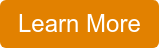Bob Bickler

# Gradient elution in liquid chromatography (HPLC, flash chromatography) is the norm. There are two typical gradient types, the linear gradient, and the step gradient. Linear gradients are mostly used due to their relative simplicity but often lack the specificity needed to purify a specific compound to a desired percentage. That is where step gradient’s improved compound targeting can be beneficial. But how do you create a step gradient?

With normal-phase flash chromatography, thin-layer chromatography (TLC) is frequently used to create purification gradients. Flash chromatography systems such as the Biotage® Selekt and Biotage® Isolera, imbedded software converts a single TLC plate’s data into a linear gradient and creates a step gradient based on the data from two TLC plates.

However, reversed-phase TLC is not always practical due to the plate’s hydrophobic surface wettability issues with highly aqueous solvents. So, reversed-phase method development and optimization then needs to be done either with HPLC or small-scale flash chromatography.

Reversed-phase linear gradient optimization is accomplished by evaluating various solvents (typically water and either methanol or acetonitrile) and gradient slopes. Step gradient method development optimization is a different story.

What I have found useful when creating a step gradient is the linear gradient elution information (target compound elution volume and % strong solvent). While there is no mathematical formula that I know of to calculate the step gradient parameters, a good rule of thumb is to increase the gradient starting % to a value about equal to a portion of the gradient distance if the compound elution volume is more than 5 CV.

For example, in the chromatogram below, a low mg portion of a 600 mg reaction mixture was purified with a 6-gram Biotage® Sfär C18 column using a 35-100% linear gradient over 10 column volumes (CV) with the product eluting at 7.9 CV, Figure 1.Figure 1. Low load linear gradient flash chromatography of a reaction mixture. The target compound eluted at 7.9 CV.

Based on this data, I decided to start the step gradient at 50%. How did I come up with this value? Well, mostly based on experience. However, looking at the data, if you consider the 7.9 CV elution volume as a fraction of the gradient distance, that becomes 7.9 CV/10 CV = 0.79. Factor in the gradient change (end % - start % or 100% - 35% = 65%) then multiply that value and the elution fraction (0.79) to get the starting gradient % (51.35%). I rounded that down to 50% for simplicity. From previous experience I have found 3 or 4 CV steps seem to work the best as a step length so I decided to hold 50% for 4 CV.

To determine the step %, again I used experience and the linear gradient data. I found that if I subtracted the elution volume, 7.9 CV, from the number of CV required to achieve the final %B (10 CV), that I could determine the step height. That value is 0.21 (10 CV - 7.9 CV = 2.1/10 = 0.21). If that fraction is multiplied by the gradient change (65%), the result would approximate the step amount (13.65%). I rounded this to 15%. So, my step gradient now is 50% for 4 CV followed by 65% for 4 CV.

In theory, this gradient should elute my target compound within the 65% step at which point the gradient can be increased to 100% to clean the column. Final gradient…

50% methanol for 4 CV

65% methanol for 4 CV

100% methanol for 2 CV

The step gradient was tested with a low load test with the 6-gram C18. The results show this step gradient provided good result though with a split product peak, something that can occur with step gradients, Figure 2.Figure 2. Step gradient test purification eluted the product within the 4CV, 65% methanol segment. The blue and red peaks are the product.

Based on this result I purified the remainder of the reaction mixture with the same gradient and a 30-gram Sfär C18 column, Figure 3.Figure 3. Reaction mixture purification with a 30-gram Sfär C18 column and step gradient.

The results show a good separation with 25% less solvent consumption (12 CV) vs. the linear gradient’s 16 CV, an added bonus.

So, when creating a reversed-phase step gradient, consider target compound retention, linear gradient start and finish %, run volume (#CV), and experiment at small scale to optimize.